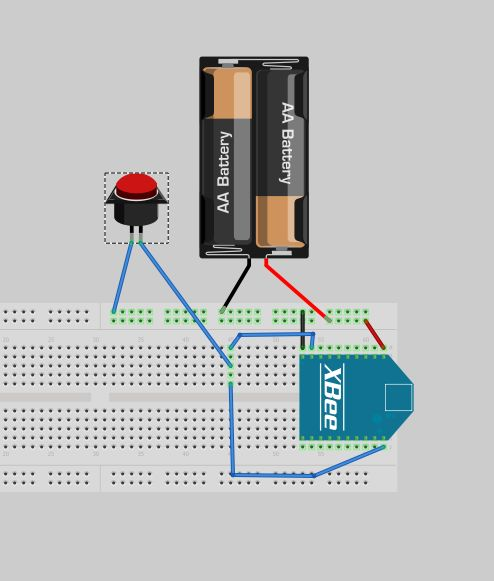# digital-in has memory?

I have a couple of xbees that are set up to sleep for about 5 minutes and pin-awake.

I have push button between ground and dio 0 and pin 8 (the pin-awake pin)

it works fine when I press the button, it awakes and send a correct message, because of switch bounce I may get a couple of messages, not a problem.

the xbee then goes back to sleep as expected.

when it wakes again in say 5 minutes, it sends the message but the message shows the D0 still 0 even though it has been 5 minutes since the last button press.

any ideas?

here is the config:

XB24-ZB_28A0.mxi
80
0
251
28A0
0
[A]ID=100
[A]SC=3FFF
[A]SD=7
[A]ZS=1
[A]NJ=FF
[A]JN=1
[A]DH=0
[A]DL=0
[A]NI=button
[A]NH=1E
[A]BH=0
[A]DD=30000
[A]NT=FF
[A]NO=0
[A]CR=3
[A]SE=E8
[A]DE=E8
[A]CI=11
[A]PL=4
[A]PM=1
[A]EE=0
[A]EO=0
[A]BD=3
[A]NB=0
[A]SB=0
[A]RO=3
[A]D7=1
[A]D6=0
[A]CT=64
[A]GT=3E8
[A]CC=2B
[A]SM=5
[A]ST=3
[A]SP=7D0
[A]SN=10
[A]SO=6
[A]PO=0
[A]D0=3
[A]D1=0
[A]D2=0
[A]D3=0
[A]D4=0
[A]D5=1
[A]P0=1
[A]P1=0
[A]P2=0
[A]PR=1FFF
[A]LT=0
[A]RP=28
[A]IR=800
[A]IC=0
[A]V+=C00

One other note is that after about two timer driven wakeups (as apposed to the pin-awake) it figures out that DIO 0 is low. It runs fine after that as long as I don’t press the button again.

Also I tried using an external pull-up resistor, same problem exists.

here is the Fritzing circuitOk if you have read and understood the previous posts. Here is a way I can get the problem to go a way or as I suspect “hide the problem”.

By changing the SO value from 6 to 0. This as expected sort of defeats the SN & SP features. But I still sleep bout for only ~1.5 minutes and not the ~5 minutes I wanted

SO option description
0x02 - Wake for ST time on each cyclic wake (after sleeping for SN sleep periods),

0x04 - Enable extended cyclic sleep (sleep for entire SN*SP time - possible data loss).

Sounds more like a pull-up problem. I see you have PR=1FFF, which means that both DIO0 and DIO9 should be high.

Are you sure this isn’t a race condition, where the Xbee wakes BEFORE the pull-ups are active? Try setting the WH=50 to delay the data sample for 50msec after ‘wake up’.

Thanks

I use X-CTU and it does not have a option for WH so I edited the above config file and added [A]WH=50. Does this work?

After the above change (if it worked) I still one or two spurious D0=0 messages

I don’t think it is a race condition because it is not intermittent. Every time I pull D9 & D0 low with the button I get a valid message showing D0 = 0 and then I always get one or two false D0 = 0 later when it wakes after the ~5 minute sleep. Could you explain the race condition you suspect?

I may be mistaken, but I think to reduce current leakage, the weak pull-ups on the Xbee are disabled during sleep. So when the Xbee wakes, for a tiny fraction of a second all inputs will ‘float’ in undetermined states.

WH would delay the reading, so it doesn’t occur at once.

You must have an older version of ZB firmware, as WH has been in there for awhile. XCTU ‘auto-learns’ settings by reading the firmware file matched to the Xbee.

You might want to try the latest firmware, which for ZigBee would be 2xA7 7 XCTU will download via the web button.

The idle pull down makes some since. But does not explain why if I never “press the button” I never get any spurious DO=0. also when SO=0 it works fine, just does not sleep for very long ~1.5 minutes.

OK, still looking for the WT, I did not see it on the X-CTU screen
my X-CTU version is 5.2.7.5

here is a default “save” for XB24-ZB version 28A7

XB24-ZB_28A7.mxi
80
0
251
28A7
0
[A]ID=0
[A]SC=FFFF
[A]SD=3
[A]ZS=0
[A]NJ=FF
[A]JN=0
[A]DH=0
[A]DL=0
[A]NI=
[A]NH=1E
[A]BH=0
[A]DD=30000
[A]NT=3C
[A]NO=0
[A]CR=3
[A]SE=E8
[A]DE=E8
[A]CI=11
[A]PL=4
[A]PM=1
[A]EE=0
[A]EO=0
[A]BD=3
[A]NB=0
[A]SB=0
[A]RO=3
[A]D7=1
[A]D6=0
[A]CT=64
[A]GT=3E8
[A]CC=2B
[A]SM=4
[A]ST=1388
[A]SP=20
[A]SN=1
[A]SO=0
[A]PO=0
[A]D0=1
[A]D1=0
[A]D2=0
[A]D3=0
[A]D4=0
[A]D5=1
[A]P0=1
[A]P1=0
[A]P2=0
[A]PR=1FFF
[A]LT=0
[A]RP=28
[A]DO=1
[A]IR=0
[A]IC=0
[A]V+=0

I have made a config change that makes the problem go away, in the configuration I put in my first post I changed

ST=3
to
ST=100

Now the question is why did this fix the problem? My theory is that the 3ms wait after waking and sending was too short for the xbee to get a ACK back so next time it woke up it sent the message again. Eventually It gets the ACK and is happy.

Anyone think this is correct or have an alternate theory?

Your theory makes sense, but is ‘pitty’ as one would think a child/enddevice would consider waiting for the parent’s ACK ‘work’ and delay powerdown until it saw the response.

You could also play with the PO setting (poll rate) because I think with PO set to 0 (default of 0x0A or 100msec) the end-device sends the data say at time zero, but doesn’t look for (poll) for the parent ACk for 100msec. So since you want to sleep fast, you should be able to push this down to perhaps 3 (30 msec) which would cause the child to pull the ACK faster. If that works you ST could be 30 (or 35 for safety)

Ok, that worked. Thanks Lynn

I now set the following

st=30
po=3

and all is well with over a 7 minute extended sleep

case closed.